Related Articles

# Seaborn | Style And Color

• Last Updated : 29 Jan, 2021

Seaborn is a statistical plotting library in python. It has beautiful default styles. This article deals with the ways of styling the different kinds of plots in seaborn.

## Seaborn Figure Styles

This affects things like the color of the axes, whether a grid is enabled by default, and other aesthetic elements.

The ways of styling themes are as follows:

• white
• dark
• whitegrid
• darkgrid
• ticks

Set the background to be white:

Given style with the help of countplot and the dataset is present in seaborn by default. load_dataset() function is used to load the dataset. set_style() function is used for plot styling.

## Python3

 `import` `seaborn as sns``import` `matplotlib.pyplot as plt` `# load the tips dataset present by default in seaborn``tips ``=` `sns.load_dataset(``'tips'``)``sns.set_style(``'white'``)` `# make a countplot``sns.countplot(x ``=``'sex'``, data ``=` `tips)`

Output: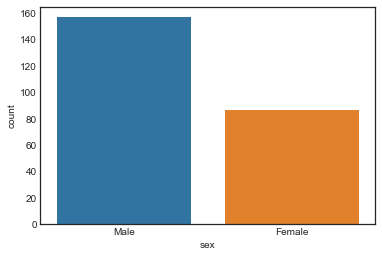Set the background to ticks:

Ticks appear on the sides of the plot on setting it as set_style(‘ticks’). palette attribute is used to set the color of the bars. It helps to distinguish between chunks of data.

## Python3

 `import` `seaborn as sns``import` `matplotlib.pyplot as plt`` ` `tips ``=` `sns.load_dataset(``'tips'``)``sns.set_style(``'ticks'``)``sns.countplot(x ``=``'sex'``, data ``=` `tips, palette ``=` `'deep'``)`

Output: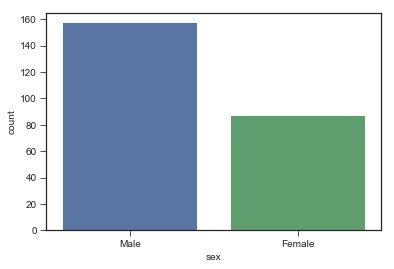Set the background to be darkgrid:

Darkgrid appear on the sides of the plot on setting it as set_style(‘darkgrid’). palette attribute is used to set the color of the bars. It helps to distinguish between chunks of data.

## Python3

 `import` `seaborn as sns``import` `matplotlib.pyplot as plt` `# load the tips dataset present by default in seaborn``tips ``=` `sns.load_dataset(``'tips'``)``sns.set_style(``'darkgrid'``)` `# make a countplot``sns.countplot(x ``=``'sex'``, data ``=` `tips)`

Output: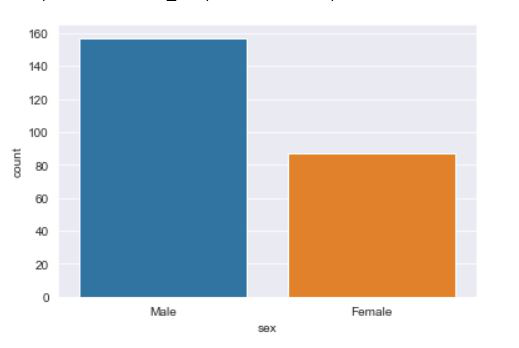Set the background to be Whitegrid:

Whitegrid appears on the sides of the plot on setting it as set_style(‘whitegrid’). palette attribute is used to set the color of the bars. It helps to distinguish between chunks of data.

## Python3

 `import` `seaborn as sns``import` `matplotlib.pyplot as plt` `# load the tips dataset present by default in seaborn``tips ``=` `sns.load_dataset(``'tips'``)``sns.set_style(``'whitegrid'``)` `# make a countplot``sns.countplot(x ``=``'sex'``, data ``=` `tips)`

Output: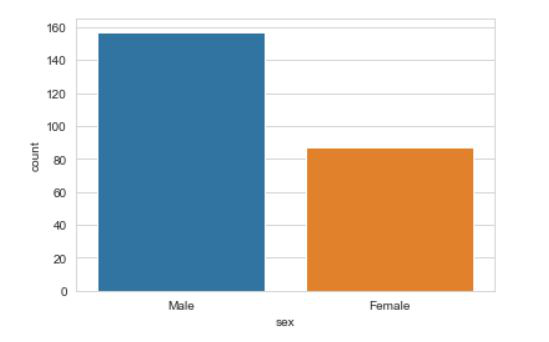## Removing Axes Spines

The despine() is a function that removes the spines from the right and upper portion of the plot by default. sns.despine(left = True) helps remove the spine from the left.

## Python3

 `import` `seaborn as sns``import` `matplotlib.pyplot as plt` `tips ``=` `sns.load_dataset(``'tips'``)``sns.countplot(x ``=``'sex'``, data ``=` `tips)``sns.despine()`

Output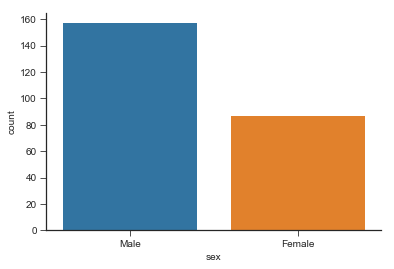## Size and aspect

Non grid plot: The figure() is a matplotlib function used to plot the figures. The figsize is used to set the size of the figure.

## Python3

 `import` `seaborn as sns``import` `matplotlib.pyplot as plt` `tips ``=` `sns.load_dataset(``'tips'``)``plt.figure(figsize ``=``(``12``, ``3``))``sns.countplot(x ``=``'sex'``, data ``=` `tips)`

Output: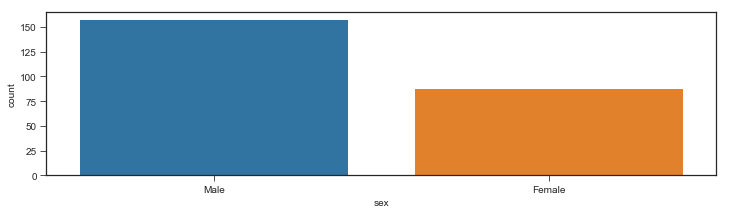Grid type plot: This example shows a regression plot of tips vs the total_bill from the dataset. lmplot stands for linear model plot and is used to create a regression plot. x =’total_bill’ sets the x axis to total_bill. y=’tip’ sets the y axis to tips. size=2 is used to the size(the height)of the plot. aspect is used to set the width keeping the width constant.

## Python3

 `import` `seaborn as sns``import` `matplotlib.pyplot as plt` `tips ``=` `sns.load_dataset(``'tips'``)``sns.lmplot(x ``=``'total_bill'``, y ``=``'tip'``, size ``=` `2``, aspect ``=` `4``, data ``=` `tips)`

Output: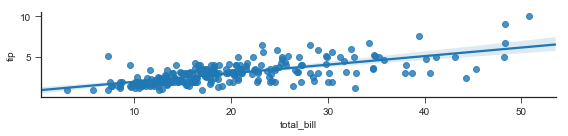## Scale and Context

The set_context() allows us to override default parameters. This affects things like the size of the labels, lines, and other elements of the plot, but not the overall style.

The context are:

• poster
• paper
• notebook
• talk

Example 1: using poster.

## Python3

 `import` `seaborn as sns``import` `matplotlib.pyplot as plt` `tips ``=` `sns.load_dataset(``'tips'``)``sns.set_context(``'poster'``, font_scale ``=` `2``)``sns.countplot(x ``=``'sex'``, data ``=` `tips, palette ``=``'coolwarm'``)`

Output: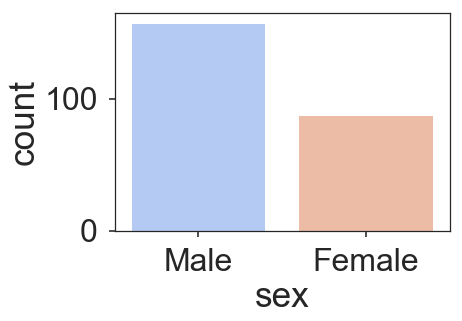Example 2: Using paper.

## Python3

 `import` `seaborn as sns``import` `matplotlib.pyplot as plt` `tips ``=` `sns.load_dataset(``'tips'``)``sns.set_context(``'paper'``, font_scale ``=` `2``)``sns.countplot(x ``=``'sex'``, data ``=` `tips, palette ``=` `'coolwarm'``)`

Output: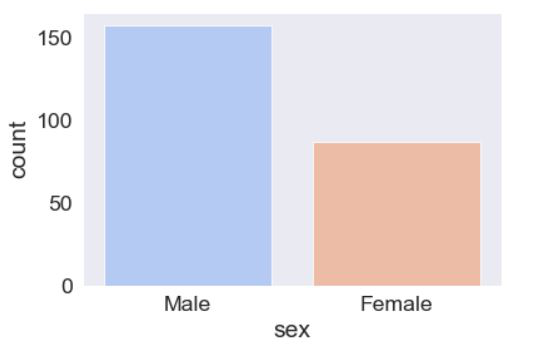Example 3: Using notebook.

## Python3

 `import` `seaborn as sns``import` `matplotlib.pyplot as plt` `tips ``=` `sns.load_dataset(``'tips'``)``sns.set_context(``'notebook'``, font_scale ``=` `2``)``sns.countplot(x ``=``'sex'``, data ``=` `tips, palette ``=``'coolwarm'``)`

Output: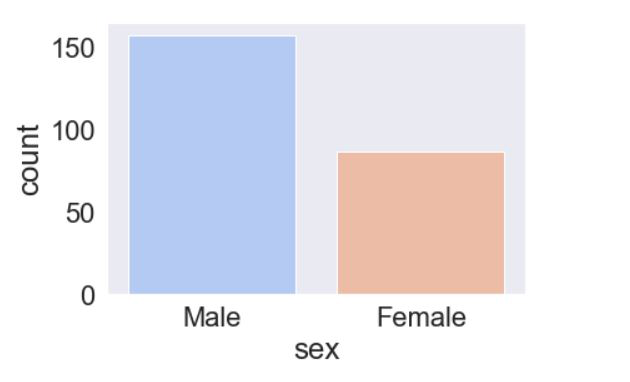Example 4: Using talk.

## Python3

 `import` `seaborn as sns``import` `matplotlib.pyplot as plt` `tips ``=` `sns.load_dataset(``'tips'``)``sns.set_context(``'talk'``, font_scale ``=` `2``)``sns.countplot(x ``=``'sex'``, data ``=` `tips, palette ``=``'coolwarm'``)`

Output: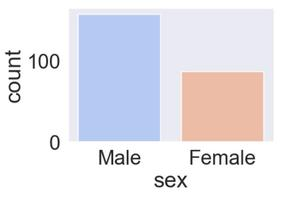Attention reader! Don’t stop learning now. Get hold of all the important Machine Learning Concepts with the Machine Learning Foundation Course at a student-friendly price and become industry ready.

My Personal Notes arrow_drop_up